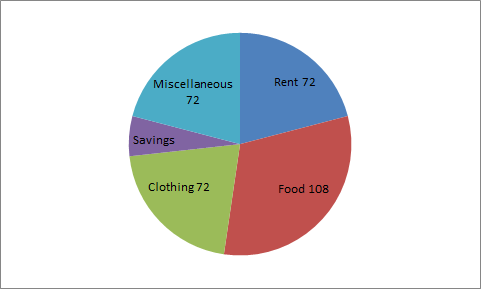# Numerical Reasoning - Numerical Reasoning Test 4

Instructions:

The following Pie diagrams shows the amounts that a family spends on food, clothing, rent, miscellaneous expenditure and savings:1:

If the annual income of the family is Rs. 60000, then the savings are:

 A. Rs. 3000 B. Rs. 6000 C. Rs. 7500 D. Rs. 9000 Answer Report Discuss Option: B Explanation : Central angle for savings = 360 - (108+72+72+72) = 36o Therefore, Savings = Rs. ( (36/360) * 60000) = Rs. 6000 Click on Discuss to view users comments. Write your comments here:

## Suggest an improvement

1. Take a free Numerical test before your next interview.
2. Take a numeracy psychometric test designed especially for CES and USB
3. Here you can practice questions for ceb numerical reasoning test.
4. These questions can be used for investment banking numerical test .
5. Here you will find questions answers for numeracy psychometric test.
6. This section provides you free online psychometric test.
7. These questions can be used for numerical assessment